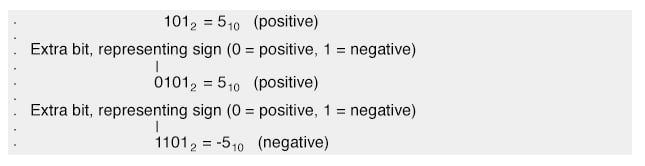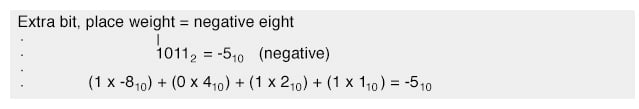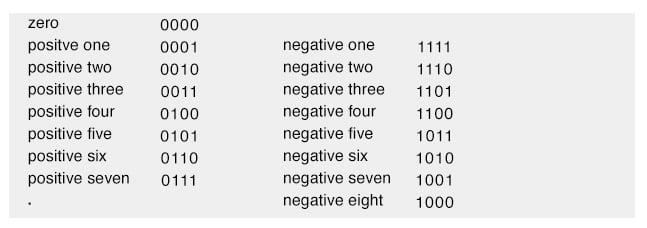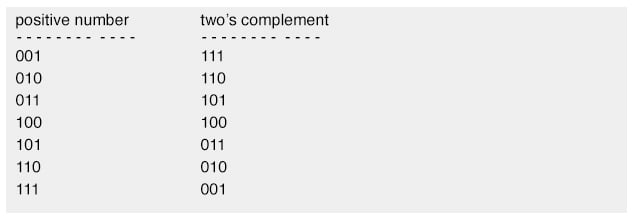# Negative Binary Numbers

## Chapter 2 - Binary Arithmetic

With addition being easily accomplished, we can perform the operation of subtraction with the same technique simply by making one of the numbers negative. For example, the subtraction problem of 7 - 5 is essentially the same as the addition problem 7 + (-5).

Since we already know how to represent positive numbers in binary, all we need to know now is how to represent their negative counterparts and we’ll be able to subtract.

Usually we represent a negative decimal number by placing a minus sign directly to the left of the most significant digit, just as in the example above, with -5. However, the whole purpose of using binary notation is for constructing on/off circuits that can represent bit values in terms of voltage (2 alternative values: either “high” or “low”).

In this context, we don’t have the luxury of a third symbol such as a “minus” sign, since these circuits can only be on or off (two possible states). One solution is to reserve a bit (circuit) that does nothing but represent the mathematical sign:As you can see, we have to be careful when we start using bits for any purpose other than standard place-weighted values. Otherwise, 11012 could be misinterpreted as the number thirteen when in fact we mean to represent negative five.

To keep things straight here, we must first decide how many bits are going to be needed to represent the largest numbers we’ll be dealing with, and then be sure not to exceed that bit field length in our arithmetic operations.

For the above example, I’ve limited myself to the representation of numbers from negative seven (11112) to positive seven (01112), and no more, by making the fourth bit the “sign” bit. Only by first establishing these limits can I avoid confusion of a negative number with a larger, positive number.

Representing negative five as 11012 is an example of the sign-magnitude system of negative binary numeration. By using the leftmost bit as a sign indicator and not a place-weighted value, I am sacrificing the “pure” form of binary notation for something that gives me a practical advantage: the representation of negative numbers.

The leftmost bit is read as the sign, either positive or negative, and the remaining bits are interpreted according to the standard binary notation: left to right, place weights in multiples of two.

#### Complementation

As simple as the sign-magnitude approach is, it is not very practical for arithmetic purposes. For instance, how do I add a negative five (11012) to any other number, using the standard technique for binary addition?

I’d have to invent a new way of doing addition in order for it to work, and if I do that, I might as well just do the job with longhand subtraction; there’s no arithmetical advantage to using negative numbers to perform subtraction through addition if we have to do it with sign-magnitude numeration, and that was our goal!

There’s another method for representing negative numbers which works with our familiar technique of longhand addition, and also happens to make more sense from a place-weighted numeration point of view, called complementation.

With this strategy, we assign the leftmost bit to serve a special purpose, just as we did with the sign-magnitude approach, defining our number limits just as before. However, this time, the leftmost bit is more than just a sign bit; rather, it possesses a negative place-weight value. For example, a value of negative five would be represented as such:With the right three bits being able to represent a magnitude from zero through seven, and the leftmost bit representing either zero or negative eight, we can successfully represent any integer number from negative seven (10012 = -810 + 12 = -710) to positive seven (01112 = 010 + 710 = 710).

Representing positive numbers in this scheme (with the fourth bit designated as the negative weight) is no different from that of ordinary binary notation. However, representing negative numbers is not quite as straightforward:Note that the negative binary numbers in the right column, being the sum of the right three bits’ total plus the negative eight of the leftmost bit, don’t “count” in the same progression as the positive binary numbers in the left column.

Rather, the right three bits have to be set at the proper value to equal the desired (negative) total when summed with the negative eight place value of the leftmost bit.

#### Two’s Complement

Those right three bits are referred to as the two’s complement of the corresponding positive number. Consider the following comparison:In this case, with the negative weight bit being the fourth bit (place value of negative eight), the two’s complement for any positive number will be whatever value is needed to add to negative eight to make that positive value’s negative equivalent.

Thankfully, there’s an easy way to figure out the two’s complement for any binary number: simply invert all the bits of that number, changing all 1’s to 0’s and vice versa (to arrive at what is called the one’s complement) and then add one! For example, to obtain the two’s complement of five (1012), we would first invert all the bits to obtain 0102 (the “one’s complement”), then add one to obtain 0112, or -510 in three-bit, two’s complement form

Interestingly enough, generating the two’s complement of a binary number works the same if you manipulate all the bits, including the leftmost (sign) bit at the same time as the magnitude bits. Let’s try this with the former example, converting a positive five to a negative five, but performing the complementation process on all four bits.

We must be sure to include the 0 (positive) sign bit on the original number, five (01012). First, inverting all bits to obtain the one’s complement: 10102. Then, adding one, we obtain the final answer: 10112, or -510 expressed in four-bit, two’s complement form.

It is critically important to remember that the place of the negative-weight bit must be already determined before any two’s complement conversions can be done. If our binary numeration field were such that the eighth bit was designated as the negative-weight bit (100000002), we’d have to determine the two’s complement based on all seven of the other bits.

Here, the two’s complement of five (00001012) would be 11110112. A positive five in this system would be represented as 000001012, and a negative five as 111110112.

RELATED WORKSHEETS:

• Share
Published under the terms and conditions of the Design Science License